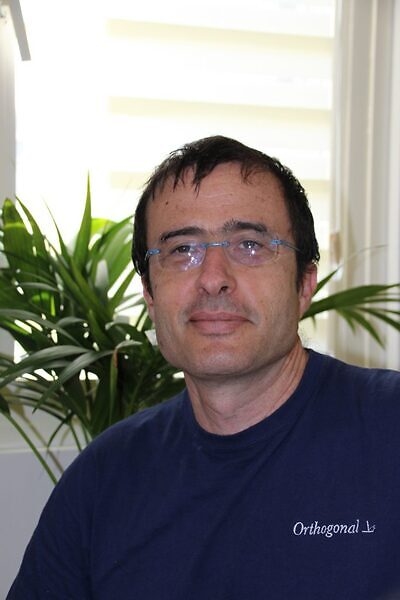# FALL 2021 Nonlinear Analysis Seminar Series### Date

2021年11月2日 (火) 16:00 17:00

Online via Zoom

### Title: Minimizers of a variational problem for nematic liquid crystals with variable degree of orientation in two dimensions

We study the asymptotic behavior, when $$k \to \infty$$, of the minimizers of the energy \begin{equation*} G_k(u)=\int_{\Omega}\Big((k-1)|\nabla|u||^2+|\nabla u|^2\Big)\,, \end{equation*} over the class of maps $$u\in H^1(\Omega,{\mathbb R}^2)$$ satisfying the boundary condition $$u=g$$ on $$\partial\Omega$$, where $$\Omega$$ is a smooth, bounded and simply connected domain in $${\mathbb R}^2$$ and $$g:\partial\Omega\to S^1$$. The motivation comes from a simplified version of Ericksen model for nematic liquid crystals. We will present similarities and differences with respect to the analog problem for the Ginzburg-Landau energy. Based on a joint work with Dmitry Golovaty.